Next: Minimization in One Up: Theory: Optimization Methods Previous: Theory: Optimization Methods

## Introduction

In the above we introduced functions of the form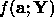which measure the fit of a model instance with n parameters a to some set of data Y. We are interested in the optimal choice of parameters, those which give the best fit to the data. This involves finding the optimum (maximum or minimum) of the functionwith respect to a. For notational simplicity we will use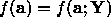. Since any maximum of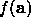is a minimum of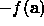we will only consider minimization. Formally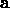is a minimum point ofif there exists a region about a of radiussuch that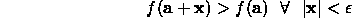The maxima and minima of a function can either be global (the highest or lowest value over the whole region of interest) or local (the highest or lowest value over some small neighbourhood). We are usually most interested in finding the global optimum (such as the model parameters which give the best match to some image data), but this can be very difficult. Often a problem will have many local optima (perhaps caused by image noise or clutter) which means that locating the single global optima can be tricky.

The most suitable methods to locate minima depend upon the nature of the function we are dealing with. There are two broad classes of algorithms.

• Local minimizers that, given a point in a `valley' of the function, locate the lowest point on the valley.
• Global minimizers that range over a region of parameter space attempting to find the bottom of the deepest valley.

If a good estimate of the position of the minimum exists we need only use a local minimizer to improve it and find the optimum choice of parameters. If no such estimate exists some global method must be used. The simplest would be to generate a set of possible start points, locally optimize each and choose the best. However, this may not be the most efficient approach.

Often an application will require both local and global methods. For instance, in a tracking problem initializing a model on the first frame may require a global search, but subsequent frames would only require a local search about the current best estimate.

The choice of which local minimization technique to use will depend upon

• Whether a is one or many-dimensional,
• Whethercan be differentiated efficiently,
• How noisyis.

In the following we will give an overview of some of the methods for locating both global and local minima. For a more comprehensive survey, including algorithmic details, see .Next: Minimization in One Up: Theory: Optimization Methods Previous: Theory: Optimization Methods

Bob Fisher
Fri Mar 28 14:12:50 GMT 1997